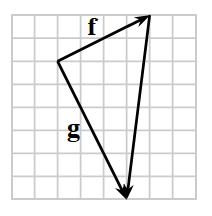### Home > APCALC > Chapter 9 > Lesson 9.3.2 > Problem9-103

9-103.

On the resource page, draw $\mathbf{c-d}$ and $\mathbf{d-c}$.

1. What one word describes the relationship between $\mathbf{c-d}$ and $\mathbf{d-c}$?

How is $5 – 3$ related to $3 – 5$?

2. Draw $\mathbf{f}$ and $\mathbf{g}$ with a third vector from the head of $\mathbf{f}$ to the head of $\mathbf{g}$ as shown at right. Label the third vector using $\mathbf{f}$ and $\mathbf{g}$.

Look at the diagram. Switch the initial and end points of vector $\mathbf{f}$.